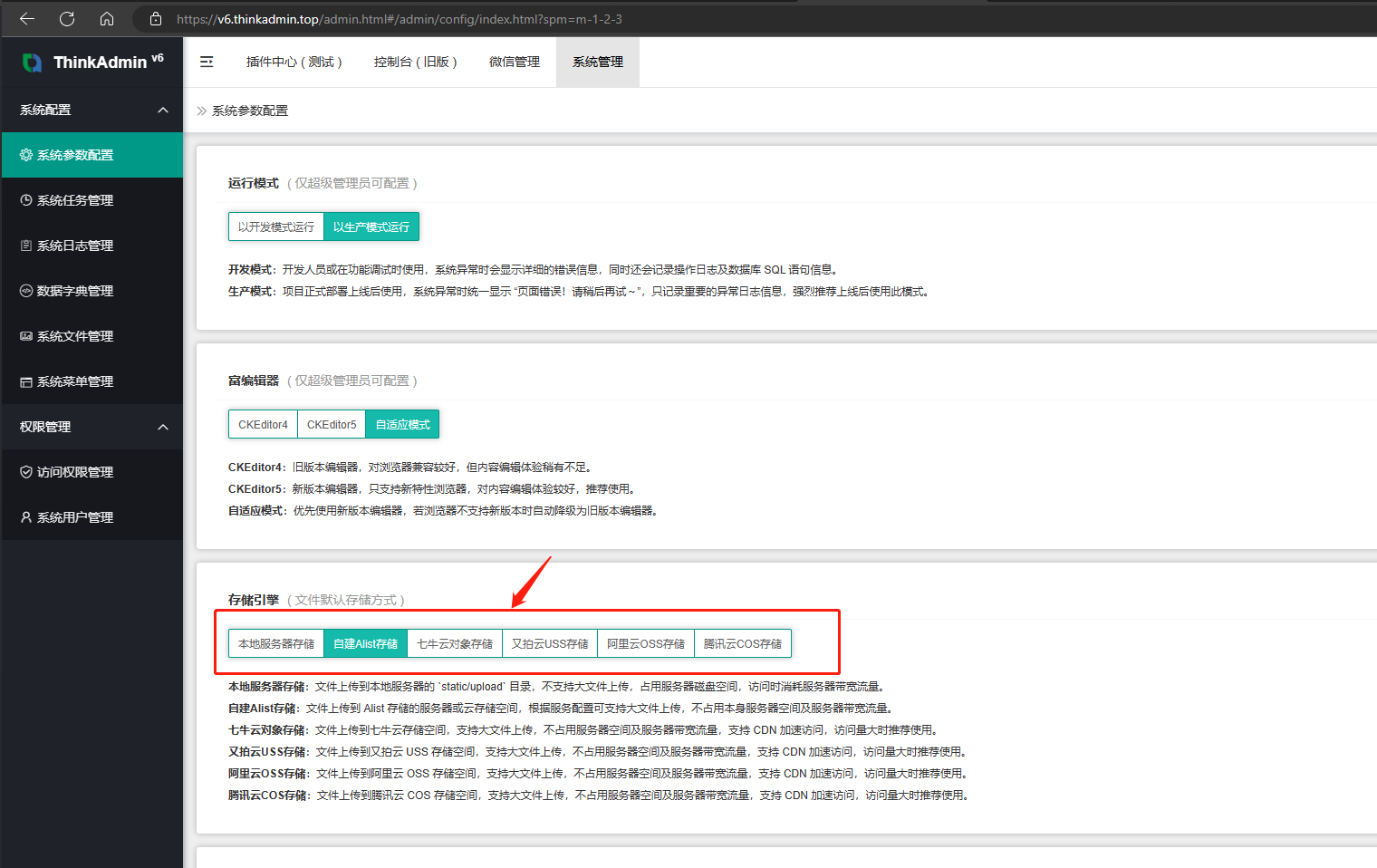# # 文件存储引擎

• 支持文件秒传，当文件已经上传到服务之后，再次上传同一个文件时将立即成功；
• 所有文件上传之后，将统一返回 url 可访问的链接地址，直接存储到内容即可访问；
• 更多文件规则可以在系统后台文件管理处配置参数，不同的存储方式配置不同的参数；## # 自动存储引擎

``````use think\admin\Storage;

\$content = '文件内容 ( 原文件内容，文档或二进制文件 )';
\$filename = '文件名称 ( 支持路径，相对 upload 目录 或 云存储根目录 ) ';

\$result = Storage::instance()->region(); // 存储区域
\$result = Storage::instance()->set(\$filename, \$content, \$safe); // 上传文件
\$result = Storage::instance()->get(\$filename, \$safe); // 读取文件
\$result = Storage::instance()->del(\$filename, \$safe); // 删除文件
\$result = Storage::instance()->has(\$filename, \$safe); // 判断是否存在
\$result = Storage::instance()->url(\$filename, \$safe); // 生成文件链接
\$result = Storage::instance()->info(\$filename, \$safe); // 获取文件参数
\$result = Storage::instance()->path(\$filename, \$safe); // 计算文件路径
``````

## # 指定存储引擎

``````// 本地服务器文件操作

\$content = '文件内容 ( 原文件内容，文档或二进制文件 )';
\$filename = '文件名称 ( 支持路径，相对 upload 目录 或 云存储根目录 ) ';

\$result = LocalStorage::instance()->set(\$filename, \$content, \$safe); // 上传文件
\$result = LocalStorage::instance()->get(\$filename, \$safe); // 读取文件
\$result = LocalStorage::instance()->del(\$filename, \$safe); // 删除文件
\$result = LocalStorage::instance()->has(\$filename, \$safe); // 判断是否存在
\$result = LocalStorage::instance()->url(\$filename, \$safe); // 生成文件链接
\$result = LocalStorage::instance()->info(\$filename, \$safe); // 获取文件参数
``````
``````// 自建 Alist 存储
// 该方式是自行搭建的存储，灵活私有，可使用内网存储

\$content = '文件内容 ( 原文件内容，文档或二进制文件 )';
\$filename = '文件名称 ( 支持路径，相对 upload 目录 或 云存储根目录 ) ';

\$result = AlistStorage::instance()->region(); // 存储区域
\$result = AlistStorage::instance()->set(\$filename, \$content); // 上传文件
\$result = AlistStorage::instance()->get(\$filename); // 读取文件
\$result = AlistStorage::instance()->del(\$filename); // 删除文件
\$result = AlistStorage::instance()->has(\$filename); // 判断是否存在
\$result = AlistStorage::instance()->url(\$filename); // 生成文件链接
\$result = AlistStorage::instance()->info(\$filename); // 获取文件参数
``````
``````// 阿里云 OSS 存储

\$content = '文件内容 ( 原文件内容，文档或二进制文件 )';
\$filename = '文件名称 ( 支持路径，相对 upload 目录 或 云存储根目录 ) ';

\$result = AliossStorage::instance()->region(); // 存储区域
\$result = AliossStorage::instance()->set(\$filename, \$content); // 上传文件
\$result = AliossStorage::instance()->get(\$filename); // 读取文件
\$result = AliossStorage::instance()->del(\$filename); // 删除文件
\$result = AliossStorage::instance()->has(\$filename); // 判断是否存在
\$result = AliossStorage::instance()->url(\$filename); // 生成文件链接
\$result = AliossStorage::instance()->info(\$filename); // 获取文件参数
``````
``````// 腾讯云 COS 存储

\$content = '文件内容 ( 原文件内容，文档或二进制文件 )';
\$filename = '文件名称 ( 支持路径，相对 upload 目录 或 云存储根目录 ) ';

\$result = TxcosStorage::instance()->region(); // 存储区域
\$result = TxcosStorage::instance()->set(\$filename, \$content); // 上传文件
\$result = TxcosStorage::instance()->get(\$filename); // 读取文件
\$result = TxcosStorage::instance()->del(\$filename); // 删除文件
\$result = TxcosStorage::instance()->has(\$filename); // 判断是否存在
\$result = TxcosStorage::instance()->url(\$filename); // 生成文件链接
\$result = TxcosStorage::instance()->info(\$filename); // 获取文件参数
``````
``````// 七牛云存储

\$content = '文件内容 ( 原文件内容，文档或二进制文件 )';
\$filename = '文件名称 ( 支持路径，相对 upload 目录 或 云存储根目录 ) ';